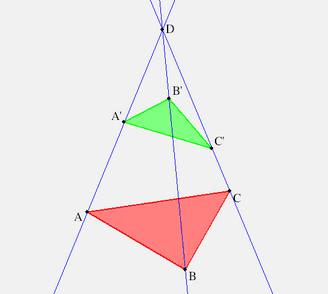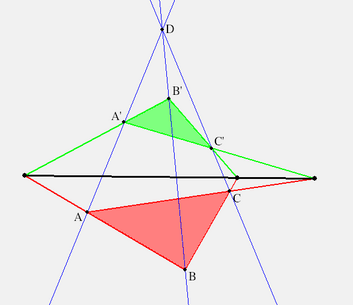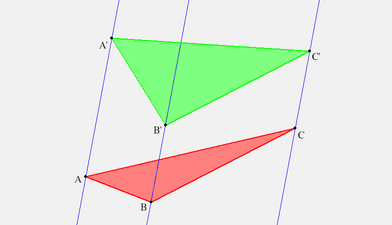## Kepler’s Ideal Lines and Points

Geometers call the set of all lines through a point a pencil of concurrent lines. Johannes Kepler (ca 1600) had the idea of extending this concept to all lines parallel to a given line as the pencil of lines concurrent at an ideal point. The stars, which for astronomer Kepler were infinitely far away, became the physical representations of the ideal points. So we also call them points at infinity. Like real (or finite) points, ideal points could lie on an ideal line insofar as the lines in each pencil are all parallel to the same (real) plane. Modern geometers prefer to define new objects in terms of sets of points.

Definition.

An ideal point $X$ is a set of lines parallel to a line. By $(\ell X)$ we shall mean that $\ell \in X$. In the plane, the set of all ideal points comprise the ideal line, $\infty$. Thus every line $\ell$ has an ideal point on itself, $X = (\ell \infty)$, and two lines are parallel if and only if they meet at infinity: $\ell || k \equiv (\ell \infty) = (k \infty)$

Question.

In space we have many ideal lines, one for each set of all mutually parallel planes. The ideal lines comprise one ideal plane, Kepler’s firmament of stars. Given two geometrical objects taken from the set of points, lines and planes, where do they meet?

Two non-parallel planes meet along a line. Two parallel planes now meet along their common ideal line. A plane and a line (that does not lie in the plane) must meet, either where the line pierces the plane. Or, if the line is parallel to the plane, they meet "at infinity", i.e. the ideal point of the line lies on the ideal line on the plane.

Two lines, however, need not meet at all, either at any real point or at any ideal point. Such lines are called skew. Can you figure out what it means for a real and an ideal line to be skew?

Kepler’s peculiar geometry had already been discovered, practically speaking, by the Rennaissance artists in their study of perspective. The horizon, particularly familiar for residents of Illinois, is an ideal line. In perspective, the horizon is drawn as a line in the painter’s canvas.

Later, these ideas were adopted by mathematicians as projective geometry. It differs from the traditional Euclidean geometry in many ways. Most notably, there is greater logical symmetry between points and lines. In projective geometry, any two points are joined by a line ( as they do in Euclid’s geometry) and any two lines (in a plane) have a point in common (non-Euclidean). In projective 3-space, any two points are joined by a line (Euclidean), and any two planes meet along line (non-Euclidean).

To exercise this expanded way of thinking we re-examine Desargues' theorem, but allowing for certain points to be ideal.

## Desargues Theorem

The figure we usually draw to illustrate Desargues Theorem looks like the following. But you should experiment with figures where the red and green triangles are in more general positions along the three lines.Click image to view desargues1.seg with KSEG.Click image to view desargues2.seg with KSEG.

Now let’s look at one ideal case, when both $D \and A^**$ are idea, i.e. $(A'A) || (B'B) || (C'C) \and (BC) || (B'C')$.Click image to view desargues3.seg with KSEG.

In this case, we have three parallal lines supporting the two triangles. C^{**}=(A'B')(AB), B^{**}=(C'A')(CA) are real (draw them !) but A^{**}=(B'C')(BC) is ideal. Now what does the conclusion of Desargues' theorem, that (A^{**}B^{**}C^{**}) is a line, mean in purely Euclidean terms? It means that A^{**} is on (B^{**}C^{**}), i.e. that (B^{**}C^{**}) is a line parallel to (both) (BC) and (B'C'). In other words, (B^{**}C^{**}) is in the pencil of (BC).

Exercise.

Easy: Give a similar description of the case that only $D$ is ideal.

Challenging: What if only $A$ is ideal?

Next, let’s play this game in reverse, and see what happens when ideal points are made real. Here is a classical theorem about parallel lines (see Tondeur, Chapter 1).

## Pappus TheoremClick image to view pappus.seg with KSEG.
Question.

Restate Pappus' theorem in Kepler’s terms.

Consider four points $W = (AC)(BD), X=(AB)(DE), Y=(BC)(EF), Z = (CD)(FA)$. The theorem says that if $W, X, Y$ are ideal, then $Z$ must also be ideal. In particular, $(XYZ) = \infty$.

Exercise.

Use KSEG or ruler and compass to investigate the case that $W$ is a real point. Is it still true? If both $X, Y$ be real too, is it still true that $(XYZ)$.

## Pascal’s Theorem in KSEG

Having extended the concept of collinearity to include ideal and real points in the plane, you should revisit Pascal’s generalization of Pappus' theorem we mentioned earlier. Pascal was so amazed by the result he discovered, he gave it a fancy name:Click image to view hexagrmmum.seg with KSEG.

The circle is a well-known conic section, as are two crossing lines. In Kepler’s sense, two parallel lines are also qualify. They are a section of a cylinder, and a cylinder is a cone with its vertex at infinity.

The intersections of the three pairs of opposite sides of a regular hexagon are ideal, and hence collinear. Inscribe a hexalateral in a circle, and see where these three points are now? Don’t be afraid to have sides of the polygon cross. Move one vertex of the hexalateral off the circle and see what happens. Finally, move all the vertices of the polygon off the circle, but carefully perserving the collinearity you’ve observed. Any conjectures about where these vertices must lie?